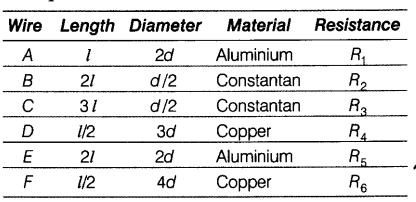# Read the following informations. (i) Resistivity of copper is lower than that of aluminium which in turn is lower than that of constantan

(i) Resistivity of copper is lower than that of aluminium which in turn is lower than that of constantan.
(ii) Six wires labelled as A, B, C, D, E and F have been designed as per the following parameters.Answer the following questions using the above data. .
(a) Which of the wires has maximum resistance and why?
(b) Which of the wires has minimum resistance and why?
(b) Wire E’has the minimum resistance, since it has least length, maximum thickness and least resistivity. [using $R = \rho ;\frac{l}{A}$]
© $R_{3}$ > $R_{5}$ > $R_{1}$ [using relation $R = \rho ;\frac{l}{A}$ and comparison ]